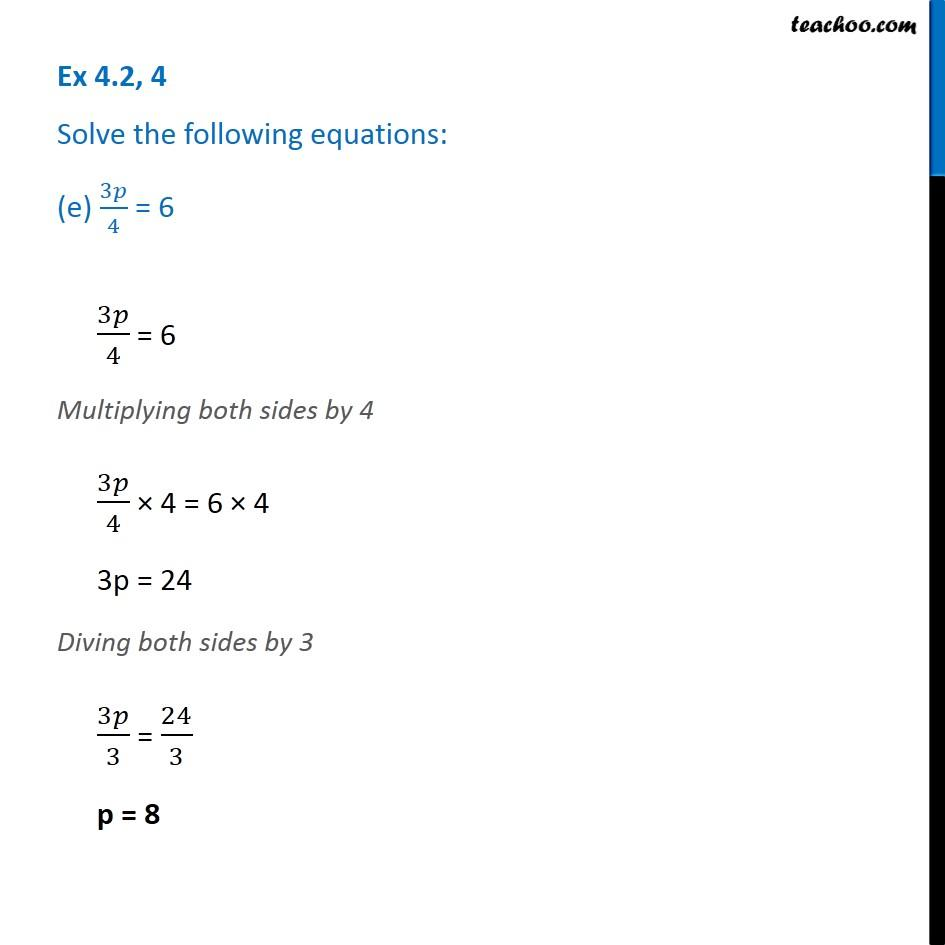Ex 4.2

Chapter 4 Class 7 Simple Equations
Serial order wiseGet live Maths 1-on-1 Classs - Class 6 to 12

### Transcript

Ex 4.2, 4 Solve the following equations: (e) 3𝑝/4 = 63𝑝/4 = 6 Multiplying both sides by 4 3𝑝/4 × 4 = 6 × 4 3p = 24 Diving both sides by 3 3𝑝/3 = 24/3 p = 8